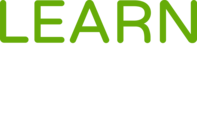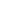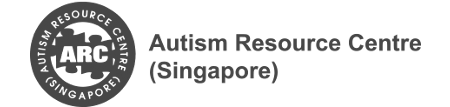#Mathematics for Life is a Course

## Mathematics for Life

Started 3 Nov 2017

### Full course description

This course introduces learners to basic mathematics concepts explained using video demonstrations.

This course is organised into four domains:

1. Numbers and Algebra
2. Measurement and Geometry
3. Statistics
4. Mathematical Processes

Students can master mathematical concepts and solve mathematical problems by engaging in hands-on learning experiences using concrete objects and visual representations of mathematical concepts.

At the end of this course, we will learn how to:

• Master the key mathematical concepts using concrete objects and visual representations
• Solve mathematical problems using numbers and symbols

This is an online course for Pathlight School students. To take this course, you’ll need to be a student of Pathlight School.

Pathlight School students can enrol in this course after logging in to Learn for Life eCampus.## Dr Yeap Ban Har

Director of Curriculum, Pathlight School

### Offered Byacademics, academic-bridging, math, mathematics, addition, making-ten, multiplication, multi-digit-calculations, fractions, decimals, subtraction, double, multiply, whole-numbers, number-operations, primary, lower-primary, upper-primary basic,